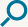# Let it be

Höfundur lags: Höfundur texta: Flytjandi: Sent inn af: Anonymous
[C]    [G]    [Am]    [F]
[C]    [G]    [F]    [C/E]    [Dm7]    [C]
When I [C]find myself in [G]times of trouble,
[Am]Mother Mary [F]comes to me,
[C]Speaking words of [G]wisdom,
let it [F]be. [C/E]    [Dm7]    [C]

And [C]in my hour of [G]darkness,
She is [Am]standing right in [F]front of me,
[C]Speaking words of [G]wisdom,
let it [F]be. [C/E]    [Dm7]    [C]

Let it [Am]be, let it [G]be,
let it [F]be, let it [C]be.
Whisper words of [G]wisdom,
let it [F]be. [C/E]    [Dm7]    [C]

And [C]when the broken [G]hearted people
[Am]Living in the [F]world agree,
[C]There will be an [G]answer,
let it [F]be. [C/E]    [Dm7]    [C]

For [C]though they may be [G]parted,
There is [Am]still a chance that [F]they will see,
[C]There will be an [G]answer,
let it [F]be. [C/E]    [Dm7]    [C]

Let it [Am]be, let it [G]be,
let it [F]be, let it [C]be.
there will be an [G]answer,
let it [F]be. [C/E]    [Dm7]    [C]

Let it [Am]be, let it [G]be,
let it [F]be, let it [C]be.
Whisper words of [G]wisdom,
let it [F]be. [C/E]    [Dm7]    [C]

[F]    [C/E]    [Dm7]    [C]    [Bb]    [F/A]    [G]    [F]    [C]
[F]    [C/E]    [Dm7]    [C]    [Bb]    [F/A]    [G]    [F]    [C]

[C]    [G]    [Am]    [F]
[C]    [G]    [F]    [C/E]    [Dm7]    [C]
[C]    [G]    [Am]    [F]
[C]    [G]    [F]    [C/E]    [Dm7]    [C]

Let it [Am]be, let it [G]be,
let it [F]be, let it [C]be.
Whisper words of [G]wisdom,
let it [F]be.  [C]    [Dm7]    [C]

And [C]when the night is [G]cloudy,
There is [Am]still a light that [F]shines on me,
[C]Shine until to[G]morrow,
let it [F]be. [C/E]    [Dm7]    [C]

I [C]wake up to the [G]sound of music,
[Am]Mother Mary [F]comes to me,
[C]speaking words of [G]wisdom,
let it [F]be. [C/E]    [Dm7]    [C]

Let it [Am]be, let it [G]be,
let it [F]be, let it [C]be.
there will be an [G]answer,
let it [F]be. [C/E]    [Dm7]    [C]

Let it [Am]be, let it [G]be,
let it [F]be, let it [C]be.
there will be an [G]answer,
let it [F]be. [C/E]    [Dm7]    [C]

Let it [Am]be, let it [G]be,
let it [F]be, let it [C]be.
Whisper words of [G]wisdom,
let it [F]be. [C/E]    [Dm7]    [C]

[F]    [C/E]    [Dm7]    [C]    [Bb]    [F/A]    [G]    [F]    [C]

When I find myself in times of trouble,
Mother Mary comes to me,
Speaking words of wisdom,
let it be.

And in my hour of darkness,
She is standing right in front of me,
Speaking words of wisdom,
let it be.

Let it be, let it be,
let it be, let it be.
Whisper words of wisdom,
let it be.

And when the broken hearted people
Living in the world agree,
There will be an answer,
let it be.

For though they may be parted,
There is still a chance that they will see,
There will be an answer,
let it be.

Let it be, let it be,
let it be, let it be.
there will be an answer,
let it be.

Let it be, let it be,
let it be, let it be.
Whisper words of wisdom,
let it be.

Let it be, let it be,
let it be, let it be.
Whisper words of wisdom,
let it be.

And when the night is cloudy,
There is still a light that shines on me,
Shine until tomorrow,
let it be.

I wake up to the sound of music,
Mother Mary comes to me,
speaking words of wisdom,
let it be.

Let it be, let it be,
let it be, let it be.
there will be an answer,
let it be.

Let it be, let it be,
let it be, let it be.
there will be an answer,
let it be.

Let it be, let it be,
let it be, let it be.
Whisper words of wisdom,
let it be.

## Hljómar í laginu

•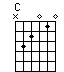•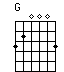•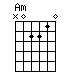••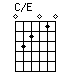••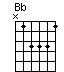•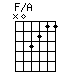•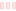Veldu hljóðfæri

Veldu nýja tóntegund

Chord data © Chordinator.com